## Examples

Example 4.2.3   Find the area enclosed by one leaf of the four-leaved rose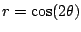.

Solution: We need the boundaries of integration. Start at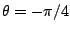and go to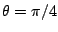. As a check, note that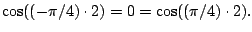We evaluate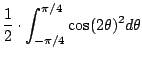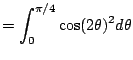(even function)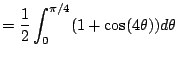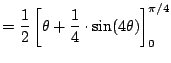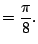We used that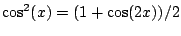and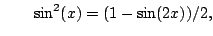(4.6)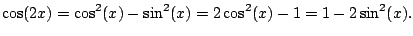Example 4.2.4   Find area of region inside the curve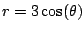and outside the cardiod curve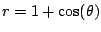.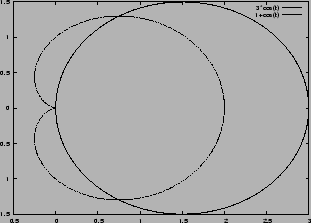Solution: This is the same as before. It's the difference of two areas. Figure out the limits, which are where the curves intersect, i.e., the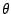such that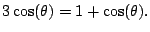Solving,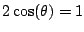, so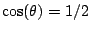, hence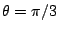and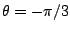. Thus the area is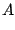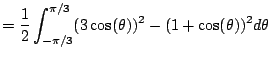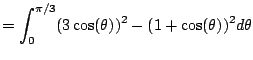(even function)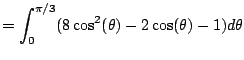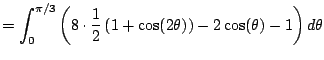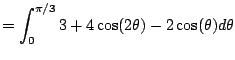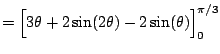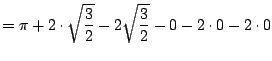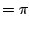William Stein 2006-03-15